Equiangular Spiral

"For problems involving directions from a fixed origin (or 'pole') O, we often find it convenient to specify a point P by its polar coordinates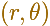whereis the distance OP and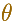is the angle that the direction OP makes with a given initial line OX, which may be identified with the x-axis of rectangular Cartesian coordinates. Of course, the pointis the same asfor any integrer n " (Coxeter, p.110)

We can use Polar coordinates for describing spirals, for example.

Coxeter introduce the Equiangular Spiral (or 'Logaritmic spiral') as the result of a continuous dilative rotation.

The polar equation of the Equiangular Spiral is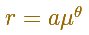It receives the name of equiangular because the angle between the radius vector and the tangent is constant.

If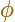is the angle between the position vector OP and the tangent at P then (in general, in polar coordinates)In the Equiangular spiral case, we can writeCalculating the derivative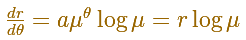Then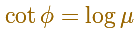The angle between the position vector OP and the tangent at P is a constant and we can write the Equiangular spiral equationThis spiral was called "spiral mirabilis" by Jacob Bernouilli.We can see that the angle formed by the radius vector and the tangent is constant.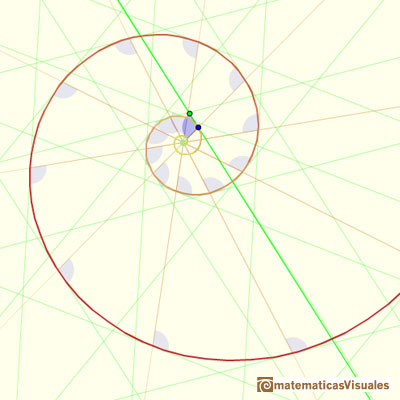In the next video you can see a particular case: the Golden Equiangular Spiral. This spiral is related with the Golden Rectangle.

/mathlet>This example is the golden equiangular spiral.

REFERENCES

Coxeter - Introduction to Geometry (John Whiley and sons)
Steinhaus - Mathematical Snapshots.
D'Arcy Thompson - On Growth and Form. (Cambridge University Press)There are infinitely many equiangular spirals through two given points.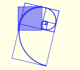The golden spiral is a good approximation of an equiangular spiral.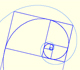Two equiangular spirals contains all vertices of golden rectangles.From Euclid's definition of the division of a segment into its extreme and mean ratio we introduce a property of golden rectangles and we deduce the equation and the value of the golden ratio.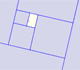A golden rectangle is made of an square and another golden rectangle.A golden rectangle is made of an square an another golden rectangle. These rectangles are related through an dilative rotation.Some properties of this platonic solid and how it is related to the golden ratio. Constructing dodecahedra using different techniques.He studied transformations of images, for example, faces.The twelve vertices of an icosahedron lie in three golden rectangles. Then we can calculate the volume of an icosahedronLeonardo da Vinci made several drawings of polyhedra for Luca Pacioli's book 'De divina proportione'. Here we can see an adaptation of the dodecahedron.